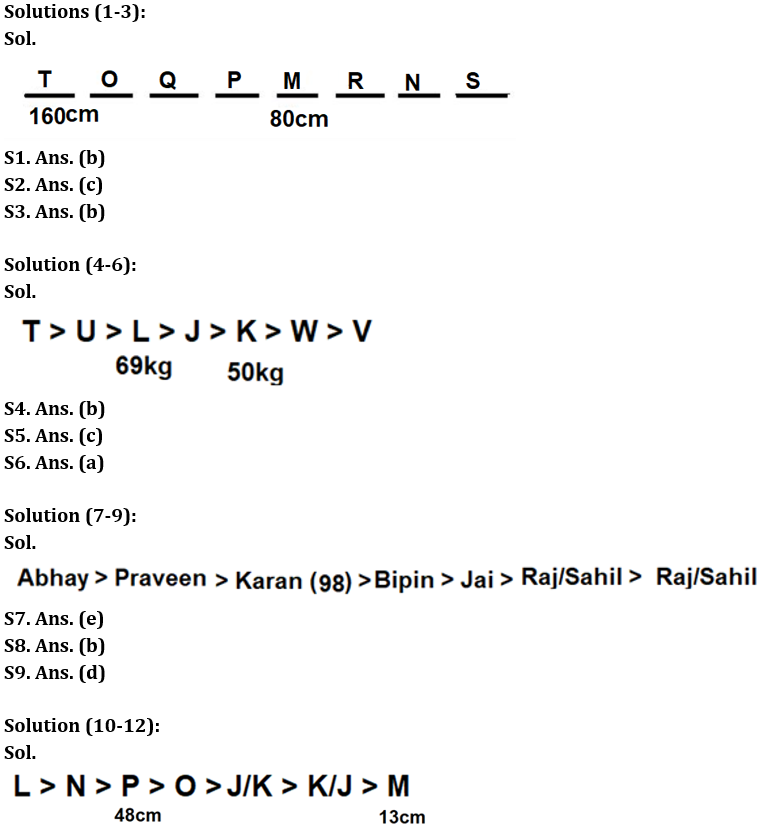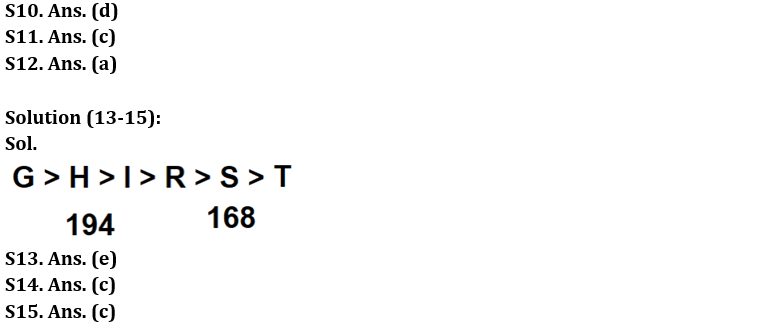Latest Banking jobs   »

# Reasoning Ability Quiz For IDBI AM/Executive 2022- 21st June

Directions (1-3): Study the following information carefully and answer the given questions.
Eight persons M, N, O, P, Q, R, S and T each have different height but not necessarily in the same order. M is taller than N but shorter than P. O is taller than P and Q. S is shorter than N. T is taller than R and O. R is taller than N but shorter than M. Q is taller than P. The height of tallest person is 160 cm and fourth shortest person is 80 cm.

Reasoning Quiz For IDBI AM/Executive 2022 21st June CHECK IN HINDI

Q1. If total height of M and N is 135 cm, then what may be the possible height of the shortest person?
(a) 65cm
(b) 45cm
(c) 55 cm
(d) Cannot be determined
(e) None of these

Q2. How many persons are taller than P?
(a) One
(b) Two
(c) Three
(d) More than four
(e) Four

Q3. Which of the following statement is true?
(a) T is the second tallest person
(b) Q is just taller than P
(c) All are true
(d) Height of R is 85cm
(e) Height of O is 160cm

Directions (4-6): Study the following information carefully and answer the given questions.
Seven persons J, K, L, T, U, V, and W each have different weights but not in the same order as given. J is not the heaviest person. W is lighter than L. Weight of neither J nor T is 69kg. T is heavier than U and V. W is not the lightest person. L is heavier than V but lighter than U. J is just heavier than K. L is not just heavier than W. Weight of J is more than 50kg. The weight of the third heaviest person is 69kg while the weight of the third lightest person is 50kg.

Q4. Who among the following is the second heaviest person?
(a) K
(b) U
(c) J
(d) T
(e) None of these

Q5. What is the possible weight of J?
(a) 75kg
(b) 72kg
(c) 55kg
(d) 48kg
(e) Cannot be determined

Q6. How many persons are heavier than K?
(a) Four
(b) Three
(c) More than four
(d) Two
(e) None of these

Directions (7-9): Study the following information carefully and answer the given questions.
Bipin, Jai, Karan, Praveen, Abhay, Raj and Sahil got different marks in Reasoning but not necessarily in the same order. Bipin got more marks than both Raj and Sahil. Karan got less marks than Praveen but more than Raj and Sahil. Jai got more marks than only 2 students. Praveen does not get the highest marks. Raj got 64 marks. The student who got the third highest marks got 98 marks. Bipin got less marks than Karan.

Q7. How many students got less marks than Sahil?
(a) None
(b) 1
(c) 2
(d) 3
(e) Can’t be determined

Q8. What may be the possible marks obtained by Abhay?
(a) 87
(b) 115
(c) 98
(d) 97
(e) 61

Q9. Which of the following statement(s) is/are definitely true?
(a) Sahil scored least marks
(b) Raj scored less than only 1 student
(c) Bipin scored less than only 2 students
(d) Raj scored less than Praveen
(e) All are true

Directions (10-12): Study the following information carefully and answer the given questions.
Amongst seven friends – J, K, L, M, N, O and P each have different heights but not in the same order as given. P is taller than O but not as tall as N. L is taller than N. J and K are shorter than O but taller than M. The height of third tallest person is 48cm and the shortest person is 13cm.

Q10. How many persons are taller than K?
(a) One
(b) Two
(c) Three
(d) Cannot be determined
(e) More than three

Q11. If total height of O and M is 55cm, then what will be the height of O?
(a) 36cm
(b) 38cm
(c) 42cm
(d) 45cm
(e) None of these

Q12. Who is just taller than P?
(a) N
(b) Either N or L
(c) J
(d) L
(e) None of these

Directions (13-15): Study the following information carefully and answer the given questions.
Six people G, H, I, R, S and T have different heights but not in the same order as given. Three people are shorter than I. More than one person is taller than R. R is taller than T and S is not the shortest. S is shorter than H who is not the tallest. The height of the second shortest is 168cm and the height of the second tallest person is 194cm.

Q13. How many people are shorter than H?
(a) Two
(b) Either two or one
(c) One
(d) Either one or four
(e) Four

Q14. What is the possible height of R?
(a) 142cm
(b) 152cm
(c) 172cm
(d) 132cm
(e) 196cm

Q15. How many people are taller than G?
(a) Three
(b) One
(c) None
(d) Two
(e) More than three

Solutions#### Congratulations!